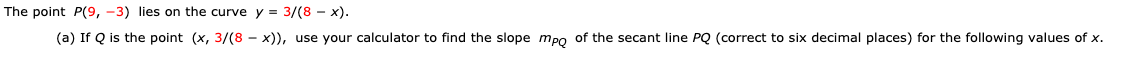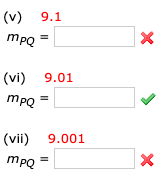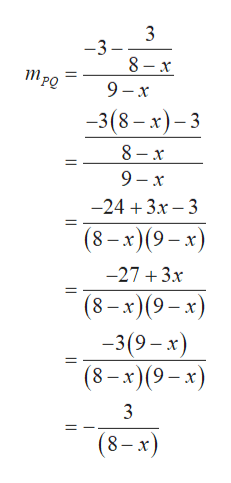# The point P(9, -3) lies on the curve y 3/(8 x)(a) If Q is the point (x, 3/(8 - x)), use your calculator to find the slope mpo of the secant line PQ (correct to six decimal places) for the following values of x (v) 9.1хТро(vi) 9.01mро(vii) 9.001хТро -

Question
13 viewshelp_outlineImage TranscriptioncloseThe point P(9, -3) lies on the curve y 3/(8 x) (a) If Q is the point (x, 3/(8 - x)), use your calculator to find the slope mpo of the secant line PQ (correct to six decimal places) for the following values of x fullscreenhelp_outlineImage Transcriptionclose(v) 9.1 х Тро (vi) 9.01 mро (vii) 9.001 х Тро - fullscreen
check_circle

Step 1

The given point P(9, –3) lies on the curve y = 3/(8 – x).

Let Q (x, 3/(8 – x)) be the point on the curve.

The slope mPQ of the secant line PQ is calculated as follows.help_outlineImage Transcriptionclose3 -3- 8 x 9 x 3(8-x)3 8 x - 9 x -243x 3 (8-x) (9-x) -27 3x (8-x)(9x) -3(9-x) (8-x)(9-x) 3 (8-x) fullscreen
Step 2

(v) The slope mPQ of the secant line PQ at the point x = 9.1 is calculated as follows.

Step 3

(vi) The slope mPQ of the secant line PQ at the point x = 9...

### Want to see the full answer?

See Solution

#### Want to see this answer and more?

Solutions are written by subject experts who are available 24/7. Questions are typically answered within 1 hour.*

See Solution
*Response times may vary by subject and question.
Tagged in

### Other